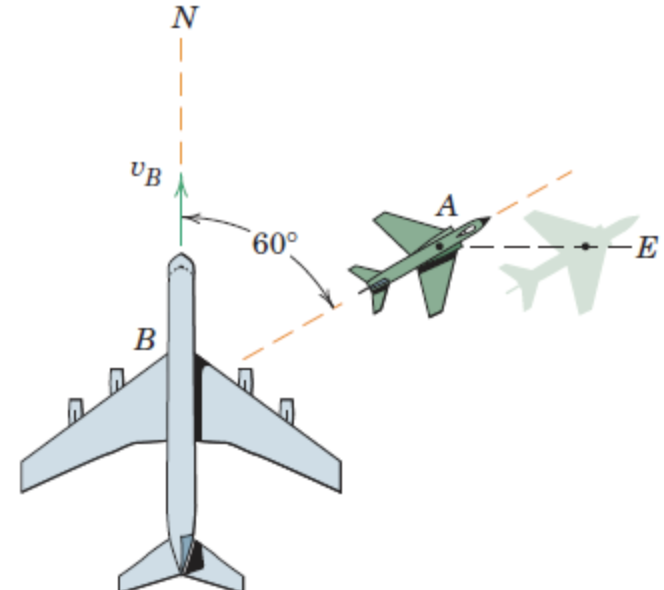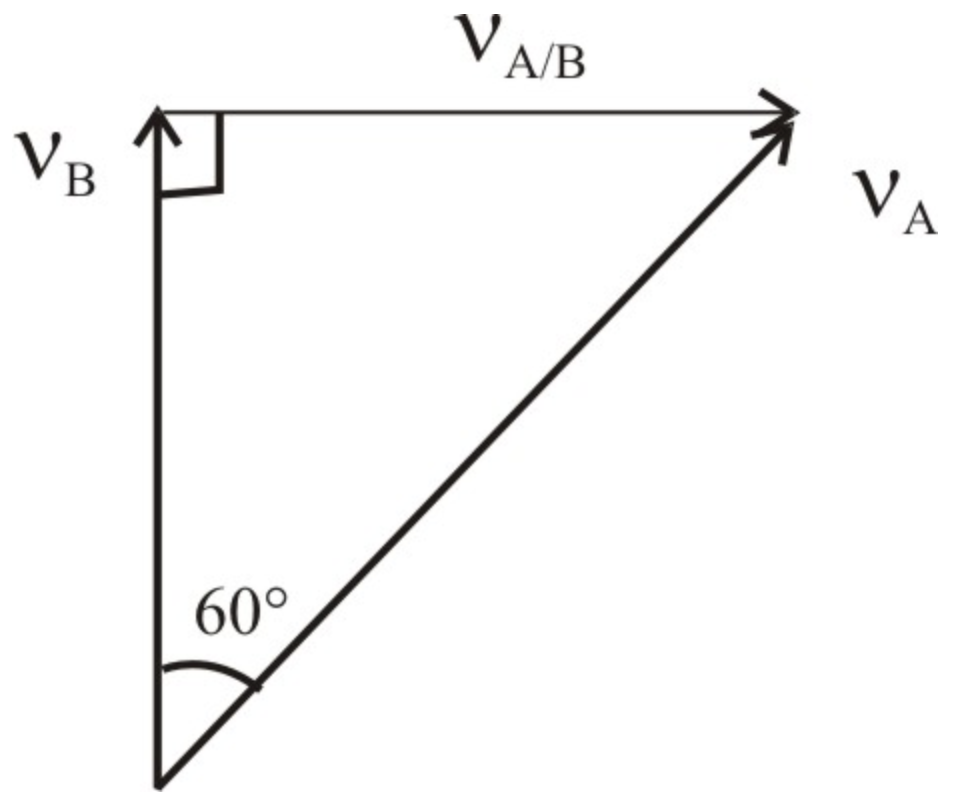##### Question

In: Mechanical Engineering

# The jet transport B is flying north with a velocity

The jet transport $$B$$ is flying north with a velocity $$v_{\mathrm{B}}=600 \mathrm{~km} / \mathrm{h}$$ when a smaller aircraft $$A$$ passes underneath the transport headed in the $$60^{\circ}$$ direction shown. To passengers in $$B$$, however, $$A$$ appears to be flying sideways and moving east. Determine the actual velocity of $$A$$ and the velocity which $$A$$ appears to have relative to $$B$$.## Solutions

##### Expert SolutionGiven:

$$v_{B}=600 \mathrm{~km} / \mathrm{h}$$

From the vector triangle,

$$v_{A}=\frac{v_{B}}{\cos 60^{\circ}}$$

$$=\frac{600}{\cos 60}$$

$$=1200$$

$$\therefore v_{A}=1200 \mathrm{~km} / \mathrm{h}$$

The velocity which A appears relative to B,

$$v_{\frac{A}{B}}=v_{B} \tan 60^{\circ}$$

$$=600 \times \tan 60^{\circ}$$

$$=1039.23$$

$$\therefore v_{\frac{A}{B}}=1039.23 \mathrm{~km} / \mathrm{h}$$

$$v_{A}=1200 \mathrm{~km} / \mathrm{h}$$

$$v_{\frac{A}{B}}=1039.23 \mathrm{~km} / \mathrm{h}$$